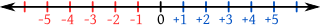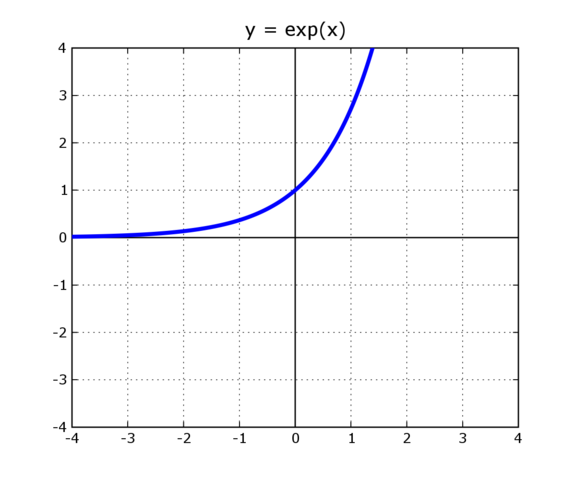# Linear Vs. Logarithmic Scales

Lesson Transcript
Instructor
Nicholas Williams

Nick has taught various subjects internationally and holds an M.Ed.

Expert Contributor
Elaine Chan

Dr. Chan has taught computer and college level physics, chemistry, and math for over eight years. Dr. Chan has a Ph.D. in Chemistry from U. C. Berkeley, an M.S. Physics plus 19 graduate Applied Math credits from UW, and an A.B. with honors from U.C .Berkeley in Physics.

This lesson defines and compares linear and logarithmic scales. We'll see how some examples and see how they are used in the real world to measure everyday objects or even earthquakes! Updated: 08/12/2020

## Defining Scale

You may have thought of a scale as something to weigh yourself with or the outer layer on the bodies of fish and reptiles. For this lesson, we're using a different definition of a scale. A scale, in this sense, is a leveled range of values and numbers from lowest to highest that measures something at regular intervals. A great example is the classic number line that has numbers lined up at consistent intervals along a line.

An error occurred trying to load this video.

Try refreshing the page, or contact customer support.

Coming up next: Interpreting Tables of Scientific Data: Practice Problems

### You're on a roll. Keep up the good work!

Replay
Your next lesson will play in 10 seconds
• 0:04 Defining Scale
• 0:31 What Is a Linear Scale?
• 1:31 What Is a Logarithmic Scale?
• 3:20 Application and Use
• 4:52 Lesson Summary
Save Save

Want to watch this again later?

Log in or sign up to add this lesson to a Custom Course.

Timeline
Autoplay
Autoplay
Speed Speed

## What Is a Linear Scale?

A linear scale is much like the number line described above. They key to this type of scale is that the value between two consecutive points on the line does not change no matter how high or low you are on it.

For instance, on the number line, the distance between the numbers 0 and 1 is 1 unit. The same distance of one unit is between the numbers 100 and 101, or -100 and -101. However you look at it, the distance between the points is constant (unchanging) regardless of the location on the line.

A great way to visualize this is by looking at one of those old school Intro to Geometry or Intermediate Algebra examples of how to graph a line. One of the properties of a line is that it is the shortest distance between two points. Another is that it is has a constant slope. Having a constant slope means that the change in x and y from one point to another point on the line doesn't change.## What Is a Logarithmic Scale?

A logarithmic scale is much different. On this scale, the value between two consecutive points not only changes, but also has a distinct pattern.

• Logarithms or 'logs' are based on exponents.
• Exponents are the 'little numbers' that are written as superscripts next to a base variable or number. For example, in the expressions 2^3 = 8, the number 3 is the exponent. These numbers multiply the base by itself a designated amount of times. In 2^3, the exponent tells us that 2 should be multiplied by itself 3 times: 2^3 = 2 x 2 x 2 = 8.

Imagine that we need to measure a really large quantity of something. Maybe minerals in soil, molecules in the air, or the intensity of sound waves, for example. Sometimes we need to create a simplified scale, where each step represents a large number of units and also increases/decreases by a certain factor.

If a scientist needs to measure billions or even trillions of molecules, they might just make a logarithmic scale with each number (i.e. from 0 to 1) increase representing an increase by a factor of 10. That would mean that going from 0 to 1 means increasing 10 units, and going from 0 to 2 means increasing 100 units, because 10^2 = 100. Numbers on a logarithmic scale are representative of a factor increase in real units.

A great way to visualize this is by looking at the graph of an exponential function. One of the properties shown in the example below is that, as x increases, y increases exponentially, or by a greater quantity for every additional unit of x.To unlock this lesson you must be a Study.com Member.
Create your account

Additional Activities

## Logarithmic Scale Questions

#### Question 1:

How would you graph the equation w = c ax on a logarithmic scale?

#### Question 2:

Why use the log-linear scale?

#### Question 3:

Does a semi-log plot help with data that spans an enormous range of values?

#### Answer 1:

To plot on a logarithmic scale, begin by taking the logarithm of both sides of the equation. The base of the logarithms can always be adjusted using the formula below:

logB A = logC A / logC B

log w = log c + log ax = log c + x log a

Make some substitutions in the notation:

y = log w ; b = log c ; m = log a

The new equation is:

y = m x + b

Does this look familiar? This is the equation of a straight line. Exponential functions plotted on a log-linear scale look like lines. The log-linear scale is also known as the semi-log plot, where one axis is a logarithmic scale, and the other is linear.

#### Answer 2:

Plotting using the log-linear scale is an easy way to determine if there is exponential growth. If there is exponential growth, you will see a straight line with slope m = log a. If the growth is slower than exponential, the curve will be concave down. If faster, it will be concave up.

#### Answer 3:

Yes. Instead of your plots trailing off to more than three pages, you will be able to see everything on one page by plotting the logarithm of the data versus the independent variable.

### Register to view this lesson

Are you a student or a teacher?

### Unlock Your Education

#### See for yourself why 30 million people use Study.com

##### Become a Study.com member and start learning now.
Back
What teachers are saying about Study.com
Create an account to start this course today
Used by over 30 million students worldwide